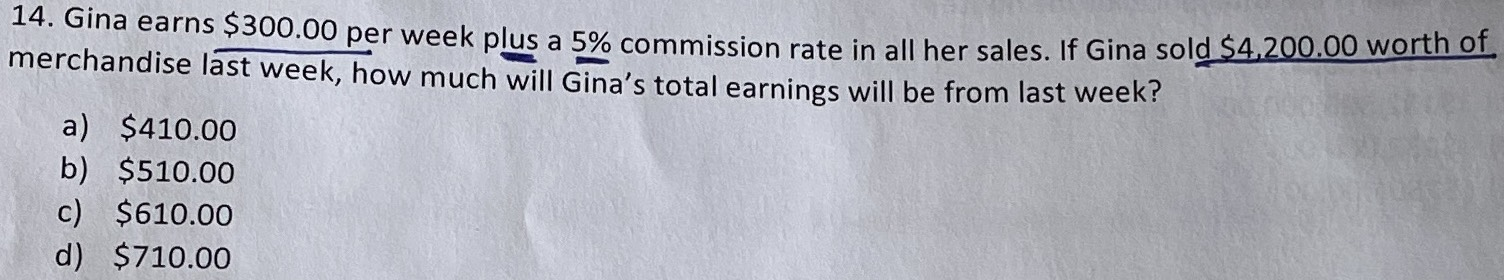### ¿Todavía tienes preguntas de matemáticas?

Pregunte a nuestros tutores expertos
Algebra
Pregunta14. Gina earns $$\ 300.00$$ per week plus a $$5 \%$$ commission rate in all her sales. If Gina sold $$\ 4,200.00$$ worth of merchandise last week, how much will Gina's total earnings will be from last week?

a) $$\ 410.00$$

b) $$\ 510.00$$

c) $$\ 610.00$$

d) $$\ 710.00$$